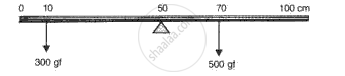Advertisement Remove all ads

# Fig. 5 Shows a Uniform Meter Scale Weighing 200 Gf. Provided at Its Centre. - Physics

Numerical

Fig. 5 shows a uniform meter scale weighing 200 gf. Provided at its centre. Two weights 300 gf and 500 gf are suspended from the ruler as shown in the diagram. Calculate the resultant torque of the ruler and hence calculate the distance from mid-point where a 100 gf should be suspended to balance the meter scale.Advertisement Remove all ads

#### Solution

Resultant torque = sum of clockwise moments  - sum of anticlockwise moments

Taking, moments about the mid point

Resulttant torque = (300 x 40) - (500 x 20)

or, Resultant torque = 12000 - 10000 = 2000 gf-cm

Let a mass of 100 gf be suspended at a distance 'd' from the mid point towards the right side,

so as to balance the metre scale.

Then, in balanced condition:

sum of clockwise moments = sum of anticlockwise moments

(300 x 40) = (500 x 20) + (100 x d)

or, 12000 = 10000 + 100d

or, 100d = 2000

or,d = 20cm to the right of the mid-point

Concept: Moment (Turning Effect) of a Force Or Torque
Is there an error in this question or solution?
Advertisement Remove all ads

#### APPEARS IN

Frank ICSE Class 10 Physics Part 2
Chapter 1 Force, Work, Energy and Power
Exercise | Q 12 | Page 57
Advertisement Remove all ads
Advertisement Remove all ads
Share
Notifications

View all notifications

Forgot password?